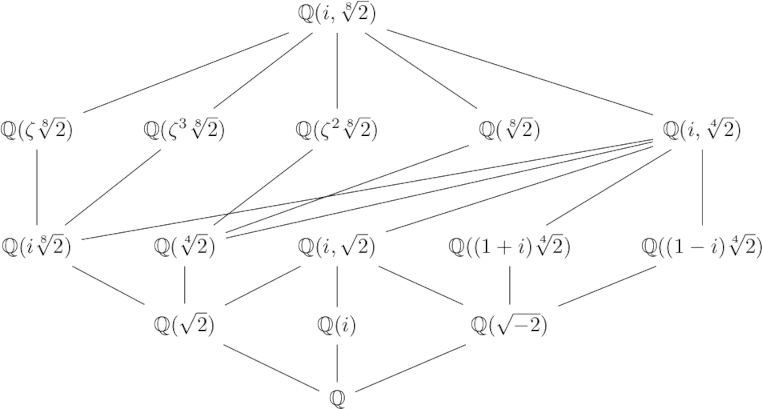# Galois connections and Galois theory

0
20What are Galois connections and what do they have to do with Galois theory?

Galois connections are much more general than Galois theory, although Galois theory provided the first and most famous example of what we now call a Galois connection.

## Galois connections

Galois connections are much more approachable than Galois theory. A Galois connection is just a pair of functions between partially ordered sets that have some nice properties. Specifically, let (A≤) and (B, ≤) be partially ordered sets. Here “≤” denotes the partial order on each set, and so could be defined differently on A and B.

We could add subscripts to distinguish the two meanings of ≤ but this is unnecessary because the meaning is always clear from context: if we’re comparing two things from Awe’re using the ≤ operator on Aand similarly for B.

Note that “≤” could have something to do with “less than” but it need not; it represents a partial order that may or may not be helpful to think of as “less than” or something analogous to less than such as “contained in.”

### Monotone and antitone functions

Before we can define a Galois connection, we need to define monotone and antitone functions.

A monotone function is an order preserving function. That is, a function f is monotone if

xyf(x) ≤ f(y).

Similarly, an antitone function is order reversing. That is, a function f is antitone if

xy ⇔ f(x) ≥ f(y).

Here ≥ is defined by

yxxy.

### Monotone and antitone connections

Galois connections have been defined two different ways, and you may run into each in different contexts. Fortunately it’s trivial to convert between the two definitions.

The first definition says that a Galois connection between A and B is a pair of monotone functions F and G such that for all a in A and b in B,

F(a) ≤ baG(b).

The second definition says that a Galois connection between A and B is a pair of antitone functions F and G such that for all a in A and b in B,

F(a) ≤ baG(b).

If you need to specify which definition you’re working with, you can call the former a monotone Galois connection and the latter an antitone Galois connection. We only need one of these definitions: if we reverse the definition of ≤ on B then a monotone connection becomes antitone and vice versa. 

How can we just reverse the meaning of ≤ willy-nilly? Recall that we said above that ≤ is just a notation for a partial order. There’s no need for it to mean “less than” in any sense, and the opposite of a partial order is another partial order.

We’ll use the antitone definition for the rest of the post because our examples are antitone. Importantly, the Fundamental Theorem of Galois Theory involves an antitone connection.

## Examples

For our first example, let A be sets of points in the plane, and let B be sets of lines in the plane. For both sets let ≤ mean subset.

For a set of points Xdefine F(X) to be the set of lines that go through all the points of X.

Similarly, for a set of lines Ydefine G(Y) to be the set of points on all the lines in Y.

Then the pair (F, G) form a Galois connection.

This example can be greatly generalized. Let R be any binary relation between A and B and let let ≤ mean subset.

Define

F(X) = x R y for all x in X

G(Y) = x R y for all y in Y

Then the pair (F, G) form a Galois connection. The example above is a special case of this construction where x R y is true if and only if x is a point on y. See .

## Galois theory

Galois theory is concerned with fields, extension fields, and automorphisms of fields that keep a subfield fixed.

I recently wrote a series of blog posts explaining what images on the covers of math books were about, and one of these posts was an explanation of the following diagram:

Each node in the graph is a field, and a line means the field on the higher level is an extension of the field on the lower level. For each graph like this of extension fields, there is a corresponding graph of Galois groups. Specifically, let L be the field at the top of the diagram and let E be any field in the graph.

The corresponding graph of groups replaces E with the group of group isomorphisms from L to L that leave the elements of E unchanged, the automorphisms of L that fix E. This map from fields to groups is half of a Galois connection pair. The other half is the map that takes each group to the field of elements of L fixed by G. This connection is antitone because if a field F is an extension of Ethen the group of automorphisms that fix F are a subgroup of the automorphisms that fix E.

***

 We could think of A and B as categories, where there is a morphism between x and y iff xy. Then a monotone Galois connection between A and B is an antitone Galois connection between A and BOp.

 Paul Taylor. Practical Foundations of Mathematics.

.

Source